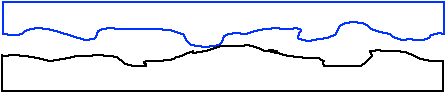#### Measuring μs

An easy way to measure the coefficient of static friction is to place two objects together and then tilt them until the top one slides. The angle at which one object starts to slip on the other is directly related to the coefficient.

When the two objects are horizontal there is no frictional force. As the objects are slowly tilted, the force of static friction must increase from zero to counteract the component of the force of gravity that acts along the interface.

Eventually, as the angle increases, that component of the force of gravity exceeds the maximum value of the force of static friction, and the top object slides off.

How does the angle at which this occurs relate to the coefficient of static friction?

Take the limiting case, the angle reached just before the block begins to slide, and draw the free-body diagram.

Here we can use:

fs = fs max = μs N

because this is the angle at which the force of static friction equals its maximum value.

Use a coordinate system with +x down the slope and +y perpendicular to the slope.

Split the force of gravity into x and y components.

Apply Newton's second law twice.
 ΣFx = m ax = 0 | ΣFy = m ay = 0 mg sin(θ) - fs = 0 | N - mg cos(θ) = 0 mg sin(θ) = μs N | N = mg cos(θ)

Substitute the second expression into the first:

mg sin(θ) = μs mg cos(θ)

The factors of mg cancel. Re-arranging gives:
 sin(θ)cos(θ)
= tan(θ) = μs

So, the coefficient of static friction is equal to the tangent of the angle at which the objects slide.

A similar method can be used to measure μk. To do that you give the top object a push as you increase the angle. When the top object keeps sliding with constant velocity, the tangent of that angle is equal to μk.

#### The microscopic view of friction

A simple model of what's happening at the microscopic level helps us understand friction. Viewed under a microscope, a surface generally looks rather rough, with hills and valleys. When you put two surfaces together, they actually make contact at very few places. When trying to move surfaces past each other, the high parts on each surface get stuck on one another.Something about the interaction between the surfaces must change when the surface area decreases. The force is certainly the same. What changes?

With the same force, decreasing the area increases the pressure, basically squishing the surfaces closer together. Things balance so the force of friction is, to a first approximation, the same.